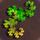## Send to a Friend# Primes and Modular Arithmetic: Do any "complete" primes exist?

Asked by PhiNotPi (12677) March 2nd, 2012

A prime P > 2 is considered “complete” if for any whole number N < P, there is another whole number K (not necessarily < P) such that:
(K^2 + K)/2 mod P = N
Does any complete prime > 2 exist?

In the above equation, (K^2 + K)/2 is the formula for the Kth Triangular number.

For a more intuitive idea of what the whole idea of prime “completeness” is, imagine the following situation:

There is a prime number of people sitting in a circle, numbered 0 through P-1.
The first person in the circle receives \$1. The 1st person to his left also gets \$1. The 2nd person to that person’s left gets \$1, then the 3rd person to his left, and so on. If the prime is complete, then every single person will eventually end up with a lot of money. If it is not complete, then there will be a person who does not get any money.

The prime 3 is not complete, as you can see below in a list of who receives money:
person 0
person 1
person 0 (person 1+2=3 that has wrapped around the circle)
person 0 (person 3+3=6)
person 1 (person 6+4=10)
etc.
You can see that the last person, person 2, never gets any money.

or

#### Using Email

Separate multiple emails with commas.
We’ll only use these emails for this message.